#There are 366 different Starters of The Day, many to choose from. You will find below some starters on the topic of Vocabulary. A lesson starter does not have to be on the same topic as the main part of the lesson or the topic of the previous lesson. It is often very useful to revise or explore other concepts by using a starter based on a totally different area of Mathematics.

Main Page

### Vocabulary Starters: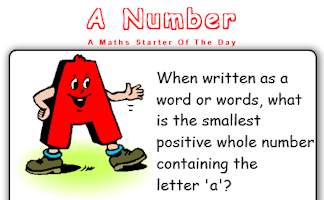When written as a word or words what is the smallest positive whole number containing the letter 'a'?A challenge to find the number which when written as a word has all the letters in alphabetical order.Can you un-jumble these mathematical words?Rearrange the letters in the expression 'Eleven Plus Two' to make another expression with the same value.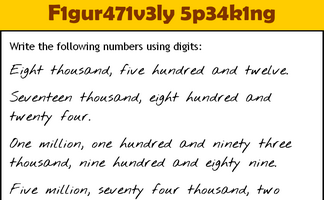Write the numbers written as words using digits.Work out the mathematical words missing from the story about buying a tent.This is the Maths version of the traditional memory game. Memorise 10 mathematical facts then recall them as they disappear from view.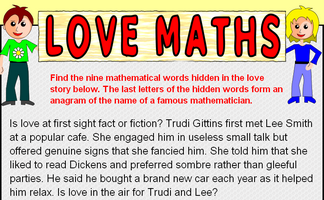Find the nine mathematical words hidden in the love story. The last letters of the hidden words form an anagram of the name of a famous mathematician.Find the words missing from the mathematical limerick.Write down as many mathematical words as possible that begin with the letter M.A small crossword puzzle containing mathematical words.Find mathematical words which can be made by using the letters in the name of your school?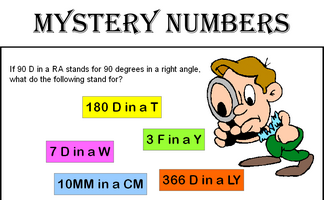Can you recognise the mystery numbers from the clues?Write down the names of all the mathematical shapes you know.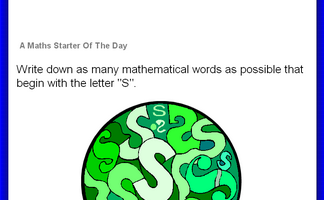Write down as many mathematical words as possible that begin with a particular letter.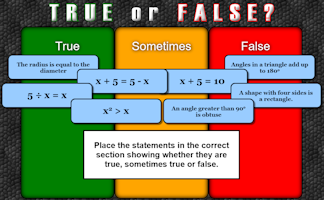An activity designed to address common misconceptions.The Vixen Value of a word is the number of vowels multiplied by the number of consonants. How many words can you find with Vixen Value of 24?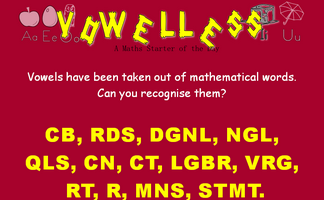Vowels have been taken out of mathematical words. Can you recognise them?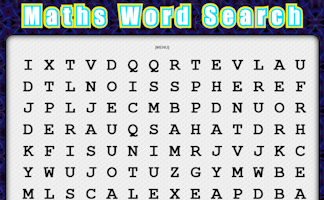Create your own mathematical word search for the whole class to enjoy.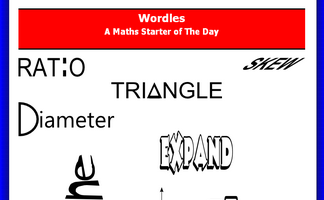Turn mathematical words into designs which will help you remember the meanings of the words.

## Exercises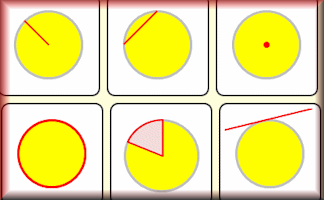#### Circle Pairs

Find the matching pairs of circle diagrams and circle properties in this interactive online game.#### Circle Parts Kim's Game

A memory game to be projected to help the whole class revise the names for the parts of a circle.#### Code Cracker

Crack the code by replacing the encrypted letters in the given text. There are lots of hints provided about code breaking techniques.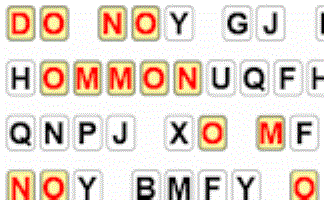#### Code Cracking Presentation

A slide presentation showing techniques for cracking simple codes and ciphers.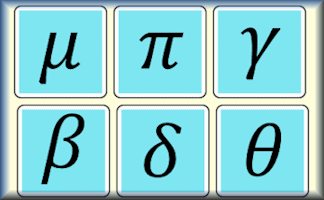#### Greek Letter Pairs

Match the names with the commonly used Greek letters.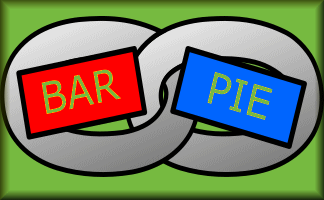#### Groups of Four

A simplified, mathematical version of the challenge seen in the British TV programme Only Connect. Find the connections between the terms.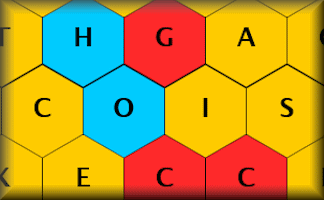#### Hexblock Hunter

The mathematical version of TV's Blockbusters game show. Find the mathematical words from the clues.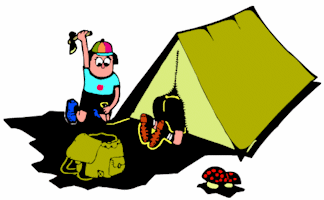#### In-tents Story

Work out the mathematical words missing from the story about buying a tent.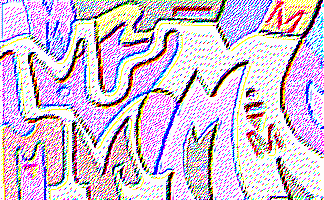#### M-Words

Here are clues to some mathematical words all beginning with the letter M. Can you figure out what they are?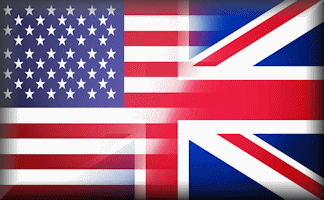#### Math vs Maths Pairs

Match the British and American words for mathematical concepts.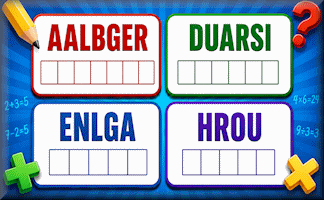#### Mathanagrams

The letters of mathematical words have been mixed up. Can you recognise them?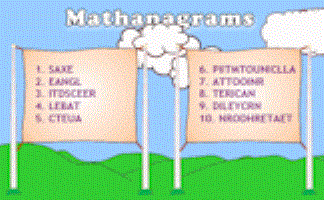#### Maths Anagrams

Jumbled words from a database of over 500 different mathematical words. Can you solve these anagrams?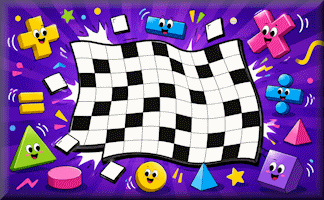#### Maths Crossword

An interactive mathematical crossword for you to do online. Find the missing words from the given clues.#### Mystery Numbers

If '7 D in a W' stands for 7 days in a week, what do you think these mystery numbers are?#### Number Rhyme

A poem about the numbers one to nine. Just for fun can you make up a last line for each verse?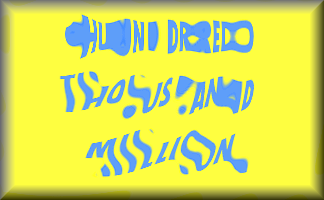#### Numbers in Words

Find the five lettered mathematical words by matching numbers with their equivalent in words.#### Polygons

Name the polygons and show the number of lines and order of rotational symmetry.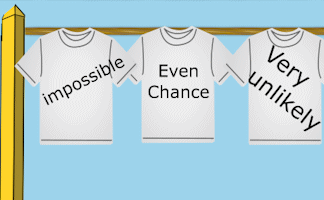#### Probability Washing Line

Hang out the washing on the line so that the probability words on the t-shirts are in order.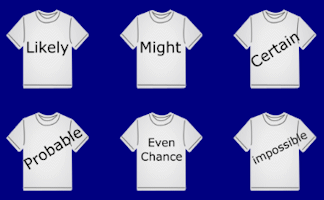#### Probability Words

A visual aid to highlight the vocabulary of probability and to debate the relationship between the given words.#### Shark's Dinner

The mathematical version of the classic hangman game. Guess the letters that are contained in the words.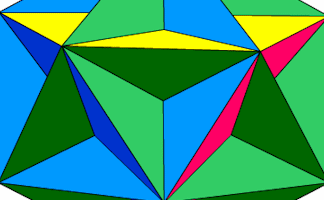#### The Great Dodecahedron

Pupils are not allowed to use their hands to point but must describe fully any shapes they can see in this picture.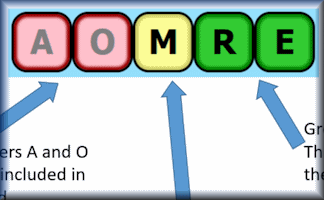#### Vocabero

Find the mathematical word from a series of guesses and clues.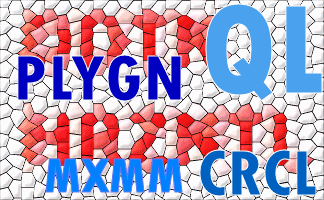#### Vowelless

Vowels have been taken out of mathematical words. Can you recognise them?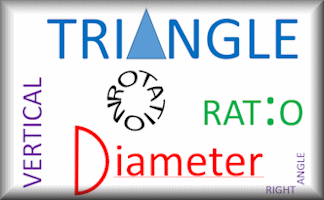#### Wordles

Remember these mathematical words for their shape and movement.#### Words and Concepts

Fill in the missing words to show an understanding of the vocabulary of equations, inequalities, terms and factors.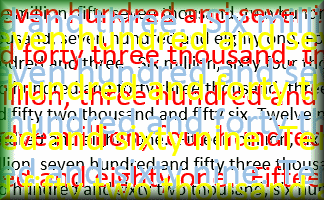#### Words in Digits

Write the numbers given in words as digits and vice versa.#### Writing Expressions

Listen to the voice saying the algebraic expression then write it in its simplest form.

### Search

The activity you are looking for may have been classified in a different way from the way you were expecting. You can search the whole of Transum Maths by using the box below.

Have today's Starter of the Day as your default homepage. Copy the URL below then select
Tools > Internet Options (Internet Explorer) then paste the URL into the homepage field.

Set as your homepage (if you are using Internet Explorer)

Do you have any comments? It is always useful to receive feedback and helps make this free resource even more useful for those learning Mathematics anywhere in the world. Click here to enter your comments.For All: